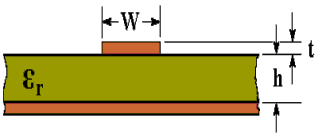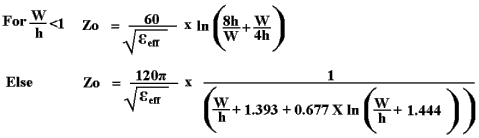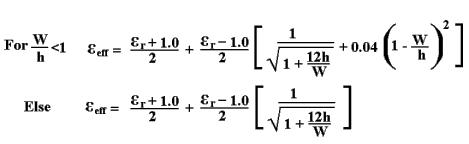# Microstrip Transmission Line Characteristic Impedance Calculator

The characteristic impedance (Zo) of a microstrip transmission line can be calculated using the active calculator or the equations at the bottom of the page.INPUT DATA Relative Dielectric Constant ( εr ): Track Width: mm Track Thickness: mm Dielectric Thickness: mm
 RESULTS Effective Dielectric Constant (εeff): Characteristic Impedance (Zo): Ohms

This calculator uses JavaScript and will function in most modern browsers. For more information see About our calculators

The following equations are from an article by Rick Hartley page 27  http://www.jlab.org/accel/eecad/pdf/050rfdesign.pdf

The equations give results within 3% of the results obtained in http://www.polarinstruments.com/support/cits/IPC1999.pdf

More calculators for transmission line can be found at http://www.circuitsage.com/tline.htmlWhere εr is the Relative Dielectric Constant

W is the Width of track

t is the Thickness of track (1 oz/ft2 = 0.035 mm)

h is the Thickness of dielectric

This calculator is provided free by Chemandy Electronics in order to promote the FLEXI-BOX